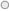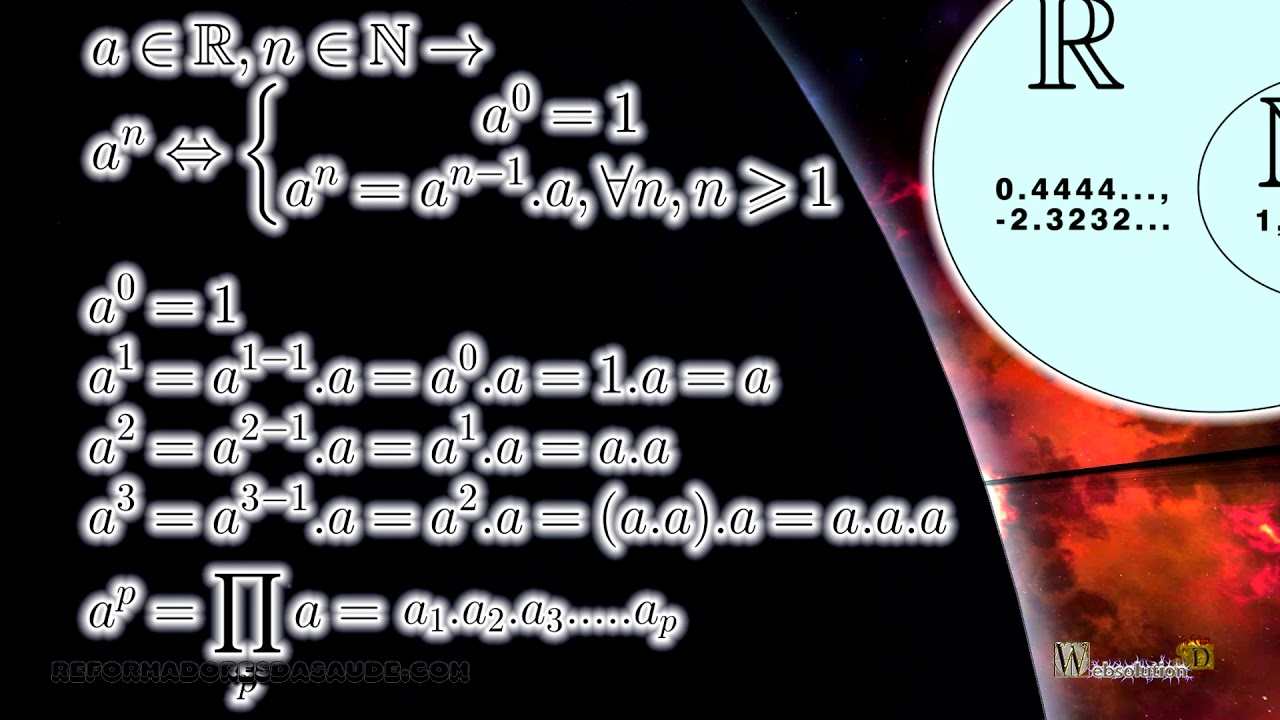## Exponentiation, Exponent Power EC1-E1100-WVITo be able to store math information to solve complex exercises, you must understand the basics of math. And for that you should not be afraid to read mathematical notation.
Check back as often as you need to learn what is being shown.
Let "a" be a number that belongs to the set of real numbers, and let "n" be a number that belongs to the naturals.
Remember that Natural is the set of nonnegative integers, in which case we take 0 as a natural number, so the number “n” belongs to this set.
And "a" belongs to the set of real numbers, which contains the natural, integer, fractional, and irrational numbers.
With these values ​​we can define that the number "a" raised to "n", when "n" is equal to zero, is equal to 1. That is any number raised to 0 equals 1. Even the 0 raised to 0 is equal to 1.
The number "a" raised to "n" is equal to "a" raised to "n" minus 1, this value multiplied by "a" for all "n", where "n" is greater than or equal to 1.
If you found it very strange, don't be afraid, keep watching.
We know that "a" raised to 0 is 1. According to the formula if "n" equals 1, we have that "a" raised to 1 equals "a" "n" minus 1, but "n" is 1, so 1 minus 1 is 0. We have "a" raised to zero, every number raised to 0 is 1. According to the formula we have to multiply it by "a", so "a" raised to 1 is "a".
And "a" raised to 2,
If we have "a" raised to 2, this is equal to "a" raised to 2 minus 1, times "a". We have 2 minus 1 is 1, so we saw that "a" raised to 1 is "a", so we have "a" times "a".
If we have "a" raised to 3, we have "a" raised to 3 minus 1, which is 2, we saw that "a" raised to 2 is "a" times "a", so "a" raised to 3 is "a "times" a "times" a ".
This gives you a logical understanding of the power of natural exponent power.
For example, if the exponent is a large number, assuming it is a value "p", then it would be equal to "a" multiplied "p" times.
If you've never seen the product, don't worry, it's not hard to understand. Do not be afraid to broaden your horizons.
Let's do some exercises.
-3 in parentheses raised to 2, according to the formula we learned, we will have 3 times 3, but the minus sign is also being raised. Just as it is even, the less times the less becomes more, the 3 times 3 is 9. So the answer is 9.
But if we have the same -3 in parentheses raised to 3, according to the formula we have the multiplication of 3 minus signs, as it is odd, less times less, times less is less, and 3 times 3, times 3 results in minus 27.
But now if we are raising without the parentheses? So the least is not being elevated, so it remains. So minus 3 raised to 2 is minus 9. And the same thing happens when minus 3 is raised to 3, minus stays because it's not being raised, and 3 times 3, times 3 results in minus 27.
If you don't remember signal multiplication, search our contents.
In the next exercise we can see 2 over 3 all raised to 3, the high multiplies the exponent of each number within the parentheses, generating 2 raised to 3 over 3 raised to 3, resulting in 8 over 27.
Unless you multiply the value of minus 2 over 3 with the value raised to 3. Unless in parentheses can be raised to 3, as 3 is odd the less remains, in the previous exercise we see that the result of the value raised to 3 is 8 over 27, as the lowest to 3 remained, it multiplies the least out of parentheses, less that multiplies less results in more, with the answer being 8 over 27.
In another we have minus 2 over 3 all of it raised to zero, now every number raised to 0 is 1.
In another exercise we have minus 1 all raised to 3532, now 3532 is even then the negative becomes positive, and the number 1 multiplied by 1 as many times results in the number 1. Remember that if the least was outside the parentheses, he would not be elevated and would remain.
In the next exercise the high number is odd, so the answer is minus 1.
In the next class we will look at other properties of exponentiation.

Exponentiation, potentiation, math, arithmetic, exercises, natural exponent